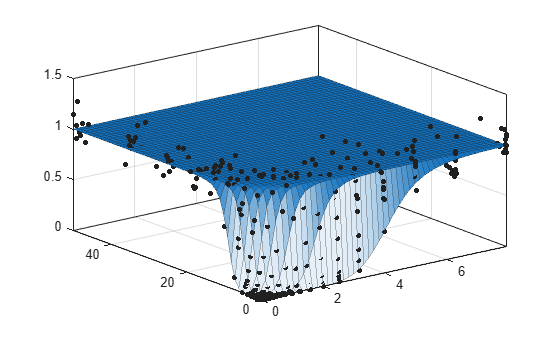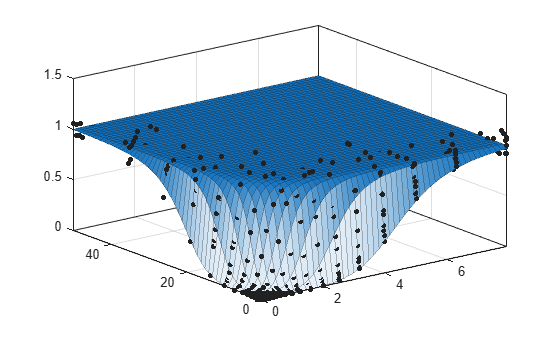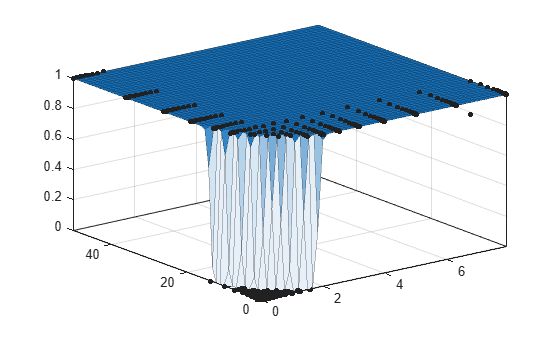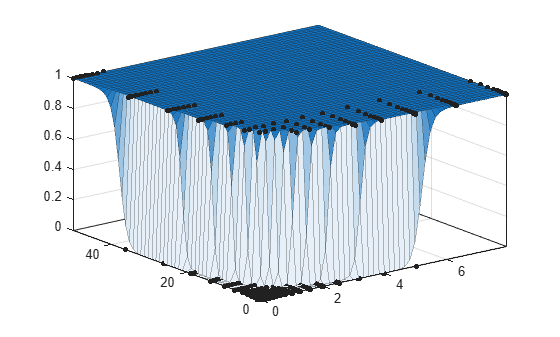Documentation

This is machine translation

Mouseover text to see original. Click the button below to return to the English version of the page.

Surface Fitting With Custom Equations to Biopharmaceutical Data

This example shows how to use Curve Fitting Toolbox™ to fit response surfaces to some anesthesia data to analyze drug interaction effects. Response surface models provide a good method for understanding the pharmacodynamic interaction behavior of drug combinations.

This data is based on the results in this paper: Kern SE, Xie G, White JL, Egan TD. Opioid-hypnotic synergy: A response surface analysis of propofol-remifentanil pharmacodynamic interaction in volunteers. Anesthesiology 2004; 100: 1373-81.

Anesthesia is typically at least a two-drug process, consisting of an opioid and a sedative hypnotic. This example uses Propofol and Reminfentanil as drug class prototypes. Their interaction is measured by four different measures of the analgesic and sedative response to the drug combination. Algometry, Tetany, Sedation, and Laryingoscopy comprise the four measures of surrogate drug effects at various concentration combinations of Propofol and Reminfentanil.

The following code, using Curve Fitting Toolbox methods, reproduces the interactive surface building with the Curve Fitting Tool described in Surface Fitting to Biopharmaceutical Data.

```data = importdata( 'OpioidHypnoticSynergy.txt' ); Propofol = data.data(:,1); Remifentanil = data.data(:,2); Algometry = data.data(:,3); Tetany = data.data(:,4); Sedation = data.data(:,5); Laryingoscopy = data.data(:,6);```

Create the Model Fit Type

You can use the `fittype` function to define the model from the paper, where `CA` and `CB` are the drug concentrations, and `IC50A`, `IC50B`, `alpha`, and `n` are the coefficients to be estimated. Create the model fit type.

```ft = fittype( 'Emax*( CA/IC50A + CB/IC50B + alpha*( CA/IC50A ) * ( CB/IC50B ) )^n /(( CA/IC50A + CB/IC50B + alpha*( CA/IC50A ) * ( CB/IC50B ) )^n + 1 )', ... 'independent', {'CA', 'CB'}, 'dependent', 'z', 'problem', 'Emax' )```
```ft = General model: ft(IC50A,IC50B,alpha,n,Emax,CA,CB) = Emax*( CA/IC50A + CB/IC50B + alpha*( CA/IC50A ) * ( CB/IC50B ) )^n /(( CA/IC50A + CB/IC50B + alpha*( CA/IC50A ) * ( CB/IC50B ) )^n + 1 ) ```

Assume `Emax = 1` because the effect output is normalized.

`Emax = 1;`

Set Fit Options

Set fit options for robust fitting, bounds, and start points.

```opts = fitoptions( ft ); opts.Lower = [0, 0, -5, -0]; opts.Robust = 'LAR'; opts.StartPoint = [0.0089, 0.706, 1.0, 0.746];```

Fit and Plot a Surface for Algometry

```[f, gof] = fit( [Propofol, Remifentanil], Algometry, ft,... opts, 'problem', Emax )```
```Success, but fitting stopped because change in residuals less than tolerance (TolFun). General model: f(CA,CB) = Emax*( CA/IC50A + CB/IC50B + alpha*( CA/IC50A ) * ( CB/IC50B ) )^n /(( CA/IC50A + CB/IC50B + alpha*( CA/IC50A ) * ( CB/IC50B ) )^n + 1 ) Coefficients (with 95% confidence bounds): IC50A = 4.149 (4.123, 4.174) IC50B = 9.045 (8.971, 9.118) alpha = 8.502 (8.316, 8.688) n = 8.288 (8.131, 8.446) Problem parameters: Emax = 1 ```
```gof = struct with fields: sse: 0.0842 rsquare: 0.9991 dfe: 393 adjrsquare: 0.9991 rmse: 0.0146 ```
`plot( f, [Propofol, Remifentanil], Algometry );`Fit a Surface to Tetany

Reuse the same `fittype` to create a response surface for tetany.

`[f, gof] = fit( [Propofol, Remifentanil], Tetany, ft, opts, 'problem', Emax )`
``` General model: f(CA,CB) = Emax*( CA/IC50A + CB/IC50B + alpha*( CA/IC50A ) * ( CB/IC50B ) )^n /(( CA/IC50A + CB/IC50B + alpha*( CA/IC50A ) * ( CB/IC50B ) )^n + 1 ) Coefficients (with 95% confidence bounds): IC50A = 4.544 (4.522, 4.567) IC50B = 21.22 (21.04, 21.4) alpha = 14.94 (14.67, 15.21) n = 6.132 (6.055, 6.209) Problem parameters: Emax = 1 ```
```gof = struct with fields: sse: 0.0537 rsquare: 0.9993 dfe: 393 adjrsquare: 0.9993 rmse: 0.0117 ```
`plot( f, [Propofol, Remifentanil], Tetany );`Fit a Surface to Sedation

`[f, gof] = fit( [Propofol, Remifentanil], Sedation, ft, opts, 'problem', Emax )`
``` General model: f(CA,CB) = Emax*( CA/IC50A + CB/IC50B + alpha*( CA/IC50A ) * ( CB/IC50B ) )^n /(( CA/IC50A + CB/IC50B + alpha*( CA/IC50A ) * ( CB/IC50B ) )^n + 1 ) Coefficients (with 95% confidence bounds): IC50A = 1.843 (1.838, 1.847) IC50B = 13.7 (13.67, 13.74) alpha = 1.986 (1.957, 2.015) n = 44.27 (42.56, 45.98) Problem parameters: Emax = 1 ```
```gof = struct with fields: sse: 0.0574 rsquare: 0.9994 dfe: 393 adjrsquare: 0.9994 rmse: 0.0121 ```
`plot( f, [Propofol, Remifentanil], Sedation );`Fit a Surface to Laryingoscopy

`[f, gof] = fit( [Propofol, Remifentanil], Laryingoscopy, ft, opts, 'problem', Emax )`
``` General model: f(CA,CB) = Emax*( CA/IC50A + CB/IC50B + alpha*( CA/IC50A ) * ( CB/IC50B ) )^n /(( CA/IC50A + CB/IC50B + alpha*( CA/IC50A ) * ( CB/IC50B ) )^n + 1 ) Coefficients (with 95% confidence bounds): IC50A = 5.192 (5.177, 5.207) IC50B = 37.77 (37.58, 37.97) alpha = 19.67 (19.48, 19.86) n = 37 (35.12, 38.87) Problem parameters: Emax = 1 ```
```gof = struct with fields: sse: 0.1555 rsquare: 0.9982 dfe: 393 adjrsquare: 0.9982 rmse: 0.0199 ```
`plot( f, [Propofol, Remifentanil], Laryingoscopy );`